• ## Python中DataFrame按照行遍历

万次阅读 多人点赞 2017-09-07 13:49:21
在做分类模型时候，需要在DataFrame中按照获取数据以便于进行训练和测试。import pandas as pddict=[[1,2,3,4,5,6],[2,3,4,5,6,7],[3,4,5,6,7,8],[4,5,6,7,8,9],[5,6,7,8,9,10]] data=pd.DataFrame(dict) print...
在做分类模型时候，需要在DataFrame中按照行获取数据以便于进行训练和测试。
import pandas as pd

dict=[[1,2,3,4,5,6],[2,3,4,5,6,7],[3,4,5,6,7,8],[4,5,6,7,8,9],[5,6,7,8,9,10]]
data=pd.DataFrame(dict)
print(data)
for indexs in data.index:
print(data.loc[indexs].values[0:-1])

实验结果：
/usr/bin/python3.4 /home/ubuntu/PycharmProjects/pythonproject/findgaoxueya/test.py
0  1  2  3  4   5
0  1  2  3  4  5   6
1  2  3  4  5  6   7
2  3  4  5  6  7   8
3  4  5  6  7  8   9
4  5  6  7  8  9  10
[1 2 3 4 5]
[2 3 4 5 6]
[3 4 5 6 7]
[4 5 6 7 8]
[5 6 7 8 9]

Process finished with exit code 0

程序员有偿接单和程序指导加QQ:734564390


展开全文• 在做分类模型时候，需要在DataFrame中按照获取数据以便于进行训练和测试。 import pandas as pd dict=[[1,2,3,4,5,6],[2,3,4,5,6,7],[3,4,5,6,7,8],[4,5,6,7,8,9],[5,6,7,8,9,10]] data=pd.DataFrame(dict) print...
• 比第一种方法快了一点，每次迭代可以直接获取每一行数据 -df.itertuples() 这种遍历方式明显快了很多 这三种遍历方式在代码量上差不多的，最后一种明显效率高啊 -apply() 如果迭代过程中的处理...
pandas的DataFrame几种遍历方式对比
我自己从数据库读了一个dataframe,df_concept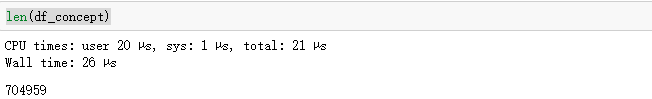df.index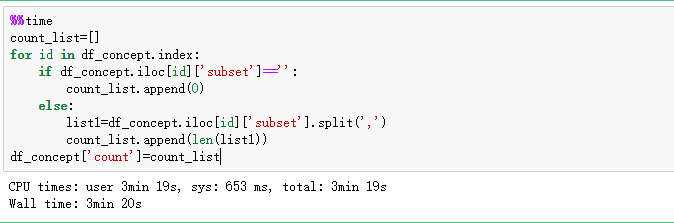df.iterrows()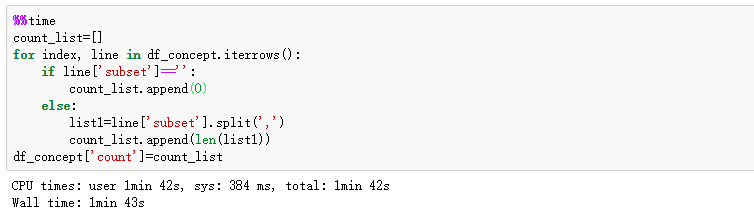比第一种方法快了一点，每次迭代可以直接获取每一行数据
df.itertuples()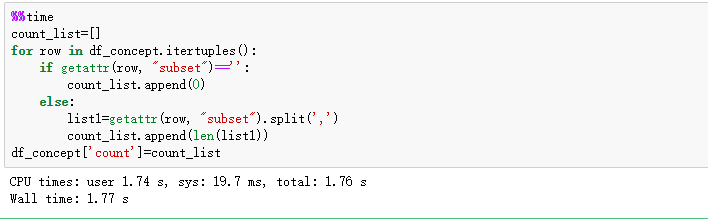这种遍历方式明显快了很多
这三种遍历方式在代码量上差不多的，最后一种明显效率高啊
apply()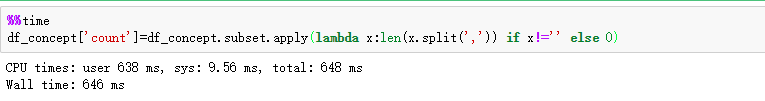如果迭代过程中的处理方法简单，通用apply的方式自定义匿名函数或者新函数效率更高，但是个人用着感觉apply的时候报错信息不太友好，很难通过报错信息定位到具体的代码行，如果处理逻辑复杂，在调试时还是不要了，或者逻辑处理好了，再用apply来跑数据更方便。


展开全文Python pandas
• 现有一个数据框pandas的dataframe: import pandas as pd inp = [{'c1':10, 'c2':100}, {'c1':11,'c2':110}, {'c1':12,'c2':120}] df = pd.DataFrame(inp) print(df) 期望输出 c1 c2 0 10 100 1 11 110 2 12 ...
现有一个数据框pandas的dataframe:

import pandas as pd
inp = [{'c1':10, 'c2':100}, {'c1':11,'c2':110}, {'c1':12,'c2':120}]
df = pd.DataFrame(inp)
print(df)

期望输出

c1   c2
0  10  100
1  11  110
2  12  120

现在我想遍历这个数据框的每一行， 在每一行当中我想通过列名访问第一行的每一个元素，例如，实现以下效果：

for row in df.rows:
print(row['c1'], row['c2'])

这里推送一个非常好用的函数：
iterrows()
这个函数同时返回 索引和行对象的生成器可以直接进行遍历

for index, row in df.iterrows():
print(row['c1'], row['c2'])

其中index遍历的是索引列的值，可以直接访问索引

展开全文• import pandas as pd dict=[[1,2,3,4,5,6],[0,0,0,0,0,0]] data=pd.DataFrame(dict) print(data) for indexs in data.index: print(data.loc[indexs].values[0:-...( 按行遍历数据) import pandas as pd dict=[...
import pandas as pd

dict=[[1,2,3,4,5,6],[0,0,0,0,0,0]]
data=pd.DataFrame(dict)
print(data)
for indexs in data.index:
print(data.loc[indexs].values[0:-1])

( 按行遍历数据)

import pandas as pd

dict=[[1,2,3,4,5,6],[0,0,0,0,0,0]]
data=pd.DataFrame(dict)
print(data)
for indexs in data.columns:
print(data[indexs])

(按列遍历数据)
展开全文• 参考链接： 遍历Pandas DataFrame中的和列 import pandas as pd dict=[[1,2,3,4,5,6],[0,0,0,0,0,0]] data=pd.DataFrame(dict) ...( 按行遍历数据) import pandas as pd dict=[[1,2,3,4,5,6],[...
• #通过iloc，loc，ix提取DataFrame中的数据 importpandasaspd dict={'name':['heji','yangyi','jiangjiming'],'age':['22','22','24'],'salary':['10000','5000','8000']} index=['a','b','c'] myframe1=pd....
• iterrows(): 按行遍历，将DataFrame的每一迭代为(index, Series)对，可以通过row[name]对元素进行访问。 itertuples(): 按行遍历，将DataFrame的每一迭代为元祖，可以通过row[name]对元素进行访问，比iterrows()...
• iterrows(): 按行遍历，将 DataFrame 的每一迭代为 (index,Series) 对，可以通过row[name] 对元素进行访问。 itertuples(): 按行遍历，将 DataFrame 的每一迭代为元组，可以通过 row[name] 对元素进行访问，比 ...python
• 在利用python进行数据分析和处理时，经常会需要遍历DataFrame行，不同的遍历方法会对数据处理的效率有很大的影响。 现在有以下的数据 import pandas as pd from pandas import DataFrame as DF from datetime ...
• 最近遇到需求要从hive表中读取数据，并且要遍历每一数据，网上找了很多资料都没有解释的很清晰的，这边记录一下。 一、主要思路 1. 首先spark读取hive表，得到DataFrame。 如果直接对spark的dataframe进行...spark
• Pandas按行遍历Dataframe的方法主要有两种：iterrows()和itertuples()，具体用法如下： # 构建数据集 import pandas as pd import numpy as np N = 20 dataset = pd.DataFrame({ 'date': pd.date_range(start='...pandas
• 表格数据中的每一或者每一列的数据结构都是Series, 它可以看成一维的表格数据, 它可以属于DataFrame的一部分也可以作为独立的数据结构存在 获取数据的值, 使用 values方法 获取索引的值, 使用 index 方法 获取每...python pandas
• iterrows(): 按行遍历，将DataFrame的每一迭代为(index, Series)对，可以通过row[name]对元素进行访问。 itertuples(): 按行遍历，将DataFrame的每一迭代为元祖，可以通过row[name]对元素进行访问，比iterrows()...
• 有时我们会对dataframe的每一行...自定义一个处理函数，并通过rdd的map函数来处理dataframe的每一行数据。 使用collect()函数 若数据量比较小，可以把数据收集到driver本地，然后再用常规的方法来遍历数据。 for rowspark实战 spark
• 文章目录取特定的列按列名选取列取特定的按行名选取按数字选取同时选取和列按名称选取按数字选取 取特定的列 按列名选取列 构造一个简单的dataframe： 按列名选取一列： df[[‘a’]]：类型为dataframe 这里...excel python pandas numpy
• 现有一个数据框pandas的dataframe: import pandas as pd inp = [{'c1':10, 'c2':100}, {'c1':11,'c2':110}, {'c1':12,'c2':120}] df = pd.DataFrame(inp) print df 期望输出 c1 c2 0 10 1...